Students can download Maths Chapter 4 Geometry Ex 4.1 Questions and Answers, Notes, Samacheer Kalvi 6th Maths Guide Pdf helps you to revise the complete Tamilnadu State Board New Syllabus, helps students complete homework assignments and to score high marks in board exams.

## Tamilnadu Samacheer Kalvi 6th Maths Solutions Term 1 Chapter 4 Geometry Ex 4.1

Question 1.
Fill in the blanks.
(i) A line through two endpoints ‘A’ and ‘B’ is denoted by ______
(ii) Aline segment from point ‘B’ to point ‘A’ is denoted by ______
(iii) A ray has ______ endpoint(s).
Solution:
(i) $$\overleftrightarrow { AB }$$
(ii) $$\bar { BA }$$
(iii) oneQuestion 2.
How many line segments are there in the given line? Name them.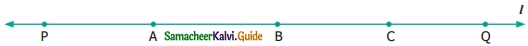Solution:
10, $$\overline { PQ }$$, $$\overline { PA }$$, $$\overline { PB }$$, $$\overline { PC }$$, $$\overline { AB }$$, $$\overline { BC }$$, $$\overline {CQ}$$, $$\overline { AQ }$$, $$\overline { BQ }$$, $$\overline { AC }$$.Question 3.
Measure the following line segments.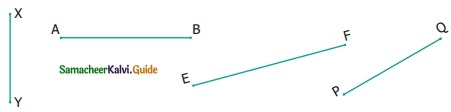Solution:
$$\overline { XY }$$ = 2.4 cm, $$\overline { AB }$$ = 3.4 cm, $$\overline { EF }$$ = 4 cm, $$\overline { PQ }$$ = 3 cm.Question 4.
Construct a line segment using a ruler and compass.
(1) $$\overline { AB }$$ = 7.5 cm
(2) $$\overline { CD }$$ = 3.6 cm
(3) $$\overline { QR }$$ = 10 cm
Solution:
(1)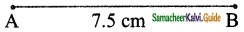(i) Draw line 1 and mark a point A on it.
(ii) Measure 7.5 cm using a compass, placing the pointer at ‘O’ and the pencil pointer at 7.5 cm.
(iii) Place the pointer of the compass at A then draw a small arc on the line 1 with the pencil pointer. It cuts line 1 at a point and name that point as B.
(iv) Now $$\overline { AB }$$ is the required line segment of length 7.5 cm.(2)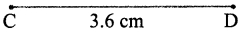(i) Draw a line 1 and mark a point C on it.
(ii) Measure 3.6 cm using a compass, placing the pointer at O and the pencil pointer at 3.6 cm.
(iii) Place the pointer of the compass at C then draw a small arc on the line 1 with the pencil pointer. It cuts line 1 at a point and names the point as D.
(iv) Now $$\overline { CD }$$ is the required line segment of length 3.6 cm.

(3)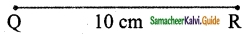(i) Draw a line 1 and mark a point Q on it.
(ii) Measure 10 cm using compass placing the pointer at O and the pencil pointer at 10 cm.
(iii) Place the pointer of the compass at Q then draw a small arc on the line 1 with the pencil pointer. It cuts the line 1 at a point and name that point as R.
(iv) Now $$\overline { QR }$$ is the required line segment of length 10 cm.Question 5.
From the given figure
(i) identify the parallel lines
(ii) identify the intersecting lines
(iii) name the points of intersection.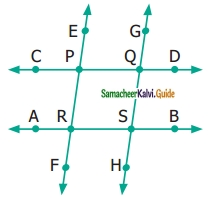Solution:
(i) Parallel lines:
(a) $$\overrightarrow{\mathrm{CD}} \text { and } \overrightarrow{\mathrm{AB}}$$
(b) $$\overrightarrow{\mathrm{EF}} \text { and } \overrightarrow{\mathrm{GH}}$$
(ii) Intersecting lines:
(a) $$\overrightarrow{\mathrm{CD}} \text { and } \overrightarrow{\mathrm{EF}}$$
(b) $$\overrightarrow{\mathrm{CD}} \text { and } \overrightarrow{\mathrm{GH}}$$
(c) $$\overrightarrow{\mathrm{AB}} \text { and } \overrightarrow{\mathrm{EF}}$$
(d) $$\overrightarrow{\mathrm{AB}} \text { and } \overrightarrow{\mathrm{GH}}$$
(iii) Point of Intersection:
P, Q, R and S are the points of Intersection.
(iii) P, Q, R and SQuestion 6.
From the given figure, name the
(i) parallel lines
(ii) intersecting lines
(iii) points of intersection.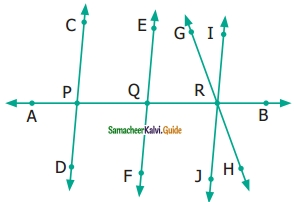Solution:
(i) $$\overleftrightarrow { CD }$$ and $$\overleftrightarrow { EF }$$, $$\overleftrightarrow { CD }$$ and $$\overleftrightarrow { IJ }$$, $$\overleftrightarrow { EF }$$ and $$\overleftrightarrow { IJ }$$
(ii) $$\overleftrightarrow { AB }$$ and $$\overleftrightarrow { CD }$$, $$\overleftrightarrow { AB }$$ and $$\overleftrightarrow { EF }$$, $$\overleftrightarrow { AB }$$ and $$\overleftrightarrow { IJ }$$, $$\overleftrightarrow { GH }$$ and $$\overleftrightarrow { IJ }$$, $$\overleftrightarrow { AB }$$ and $$\overleftrightarrow { GH }$$
(iii) P, Q and RQuestion 7.
From the given figure, name
(i) all pairs of parallel lines.
(ii) all pairs of intersecting lines.
(iii) pair of lines whose point of intersection is ‘V’.
(iv) point of intersection of the lines ‘l2‘ and ‘l3‘.
(v) point of intersection of the lines ‘l1‘, and ‘l5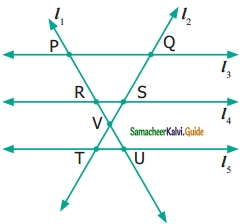Solution:
(i) Pairs of parallel lines:

• l3 and l4
• l4 and l5
• l3 and l5

(ii) Pairs of intersecting lines:

• l1 and l2
• l1 and l3
• l1 and l4
• l1 and l5
• l2 and l3
• l2 and l4
• l2 and l5

(iii) l1 and l2 intersect at ‘V’
(iv) point of intersection of the lines ‘l2‘ and; l5‘ is ‘Q’
(v) point of intersection of the lines ‘l1‘ and ‘l5‘ is ‘U’Objective Type Questions

Question 8.
The number of line segments in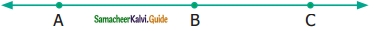(a) 1
(b) 2
(c) 3
(d) 4
Solution:
(c) 3

Question 9.
A line is denoted as __________
(a) AB
(b) $$\overrightarrow{AB}$$
(c) $$\overleftrightarrow {AB}$$
(d) $$\overline { AB }$$
Solution:
(c) $$\overleftrightarrow {AB}$$